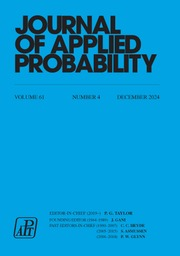Home
Hostname: page-component-6f6fcd54b-vl5pb Total loading time: 0.182 Render date: 2021-05-11T02:34:31.701Z Has data issue: true Feature Flags: {}Journal of Applied Probability

# Exponential Random Graphs as Models of Overlay Networks

Published online by Cambridge University Press:  14 July 2016

Corresponding

## Abstract

In this paper we give an analytic solution for graphs with n nodes and E = cn log n edges for which the probability of obtaining a given graph G is µn (G) = exp (- βi=1 n d i 2), where d i is the degree of node i. We describe how this model appears in the context of load balancing in communication networks, namely peer-to-peer overlays. We then analyse the degree distribution of such graphs and show that the degrees are concentrated around their mean value. Finally, we derive asymptotic results for the number of edges crossing a graph cut and use these results (i) to compute the graph expansion and conductance, and (ii) to analyse the graph resilience to random failures.

## MSC classification

Type
Research Article
Information
Journal of Applied Probability , March 2009 , pp. 199 - 220

## References

 Ball, F. and Barbour, A. (1990). Poisson approximation for some epidemic models. J. Appl. Prob. 27, 479490.CrossRefGoogle Scholar
 Besag, J. E. (1974). Spatial interaction and the statistical analysis of lattice systems. J. R. Statist. Soc. Ser. B 36, 192236.Google Scholar
 Bollobàs, B. (2001). Random Graphs (Camb. Stud. Adv. Math. 73). Cambridge Univeristy Press.CrossRefGoogle Scholar
 Brémaud, P. (1999). Markov chains, Gibbs fields, Monte Carlo Simulation, and Queues. Springer, New York.Google Scholar
 Chung, F. (1996). Laplacians of graphs and Cheeger's inequalities. In Combinatorics, Paul Erdös is Eighty, Vol. 2 (Keszthely, 1993), János Bolyai Mathematical Society, Budapest, pp. 157172.Google Scholar
 Draief, M., Ganesh, A. and Massoulié, L. (2008). Thresholds for virus spread on networks. Ann. Appl. Prob. 18, 359378.CrossRefGoogle Scholar
 Durrett, R. (2007). Random Graph Dynamics. Cambridge University Press.Google Scholar
 Erdös, P. and Rényi, A. (1960). On the evolution of random graphs. Magyar Tud. Akad. Mat. Kutató Int. Közl. 5, 1761.Google Scholar
 Frank, O. and Strauss, D. (1986). Markov graphs. J. Amer. Statist. Assoc. 81, 832842.CrossRefGoogle Scholar
 Ganesh, A. J., Kermarrec, A.-M. and Massoulié, L. (2003). Network awareness and failure resilience in self-organizing overlay networks. In Proc. IEEE Symp. Reliab. Distributed Systems, IEEE, pp. 4755.Google Scholar
 Ganesh, A. J. and Massoulié, L. (2003). Failure resilience in balanced overlay networks. In Proc. 41st Allerton Conf. Commun., Control Comput., IEEE.Google Scholar
 Ganesh, A. J., Kermarrec, A.-M. and Massoulié, L. (2003). Probabilistic reliable dissemination in large-scale systems. IEEE Trans. Parallel Distributed Systems 14, 248258.Google Scholar
 Ganesh, A. J., Massoulié, L. and Towsley, D. (2005). The effect of network topology on the spread of epidemics. In Proc. IEEE INFOCOM 2005, IEEE, pp. 14551466 Google Scholar
 Gkantsidis, C., Mihail, M. and Saberi, A. (2003). Conductance and congestion in power law graphs. In Proc. ACM SIGMETRICS 2003, ACM, pp. 148159.Google Scholar
 Holland, P. W. and Leinhardt, S. (1981). An exponential family of probability densities for directed graphs. J. Amer. Statist. Assoc. 76, 3365.CrossRefGoogle Scholar
 Janson, S. (2009). The probability that a random multigraph is simple. To appear in Combinatorics Prob. Comput. CrossRefGoogle Scholar
 Lovász, L. (1996). Random walks on graphs: a survey. In Combinatorics, Paul Erdös is Eighty, Vol. 2, (Keszthely, 1993), János Bolyai Mathematical Society, Budapest, pp. 353397.Google Scholar
 Lua, E. K. et al. (2005). A survey and comparison of peer-to-peer overlay network schemes. IEEE Commun. Surveys Tutorials 7, 7293.Google Scholar
 McDonald, D. (1979). A local limit theorem for large deviations of sums of independent, nonidentically distributed random variables. Ann. Prob. 7, 526531.CrossRefGoogle Scholar
 McKay, B. D. and Wormald, N. C. (1990). Asymptotic enumeration by degree sequence of graphs of high degree. Europ. J. Combinatorics 11, 565580.CrossRefGoogle Scholar
 Metropolis, N. et al. (1953). Equations of state calculations by fast computing machines. J. Chemical Physics 21, 10871092.CrossRefGoogle Scholar
 Mohar, B. (1997). Some applications of Laplace eigenvalues of graphs. In Graph Symmetry, eds Hahn, G. and Sabidussi, G., Kluwer, Dordrecht, pp. 225275.CrossRefGoogle Scholar
 Park, J. and Newman, M. E. J. (2004). Statistical mechanics of networks. Phys. Rev. E 70, 066117.CrossRefGoogle ScholarPubMed
 Snijders, T. A. B. (2002). Markov chain Monte Carlo estimation of exponential random graph models. J. Social Structure 3, 140.Google Scholar
 Solomonoff, R. and Rapoport, A. (1951). Connectivity of random nets. Bull. Math. Biophysics 13, 107117.CrossRefGoogle Scholar
 Steinmetz, R. and Wehrle, K. (2005). What is this peer-to-peer about? In Peer-to-Peer Systems and Applications, Springer, Berlin, pp. 916.CrossRefGoogle ScholarYou have Access

# Send article to Kindle

Note you can select to send to either the @free.kindle.com or @kindle.com variations. ‘@free.kindle.com’ emails are free but can only be sent to your device when it is connected to wi-fi. ‘@kindle.com’ emails can be delivered even when you are not connected to wi-fi, but note that service fees apply.

Find out more about the Kindle Personal Document Service.

Exponential Random Graphs as Models of Overlay Networks
Available formats
×

# Send article to Dropbox

To send this article to your Dropbox account, please select one or more formats and confirm that you agree to abide by our usage policies. If this is the first time you use this feature, you will be asked to authorise Cambridge Core to connect with your <service> account. Find out more about sending content to Dropbox.

Exponential Random Graphs as Models of Overlay Networks
Available formats
×

# Send article to Google Drive

To send this article to your Google Drive account, please select one or more formats and confirm that you agree to abide by our usage policies. If this is the first time you use this feature, you will be asked to authorise Cambridge Core to connect with your <service> account. Find out more about sending content to Google Drive.

Exponential Random Graphs as Models of Overlay Networks
Available formats
×
×